Click to Chat

1800-1023-196

+91-120-4616500

CART 0

• 0

MY CART (5)

Use Coupon: CART20 and get 20% off on all online Study Material

ITEM
DETAILS
MRP
DISCOUNT
FINAL PRICE
Total Price: Rs.

There are no items in this cart.
Continue ShoppingCircleCircle holds a high pedestal in the entire Syllabus of Co-ordinate Geometry in Mathematics. It is the easiest topic of coordinate geometry and with a bit of hard work, it becomes very easy to answer all the questions of this topic. The preliminary knowledge of the concept of Straight Lines is a prerequisite to study Circles.

In this chapter, we discuss the algebraic equations representing a circle and the lines associated with it i.e. a tangent, a pair of tangents and a chord of contact. The concepts of family of circles and that of common tangents to two circles under various configurations are given due importance.
A circle can be defined as the curve traced out by a point that moves so that its distance from a given point is constant. The distance between any of the points and the center is called the radius. It can also be defined as the locus of a point equidistant from a fixed point.

The general equation of a circle in Cartesian coordinates with centre at (a, b) and radius r is given by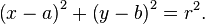If the center is at (0, 0) then the equation becomesIn parametric form it may be represented aswhere t is a parameter ranging between 0 to 2π.

We first recall the important terms and formulae related to circles:

Length of circumference in a circle is given by 2πr, where r is the radius.

The diameter is the longest chord of the circle.

Area of a circle is given by πr2.

The circle which is centered at the origin with radius 1 is called the unit circle.

A line segment whose end points lie on a circle is defined as a chord.

A line that cuts a circle at two distinct points is called a secant.

Chords are equidistant from the center of a circle if and only if they are equal in length.

Equal chords of a circle subtend equal angles at the centerAn arc is a section of the circumference of a circle.

A sector is an area of a circle bounded by 2 radii.

A quadrant is one-quarter of a circle.

A segment of a circle is the part bounded by a chord and the circumference.

Equal arcs of a circle subtend equal angles at the center.

An angle in a semi-circle is a right angle.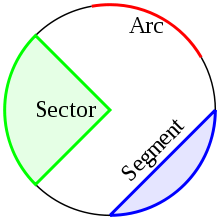Theorem:

An angle at the circumference of a circle is half the angle at the center subtended by the same arc.

Proof:

Let AB be an arc of a circle with center O, and let P be any point on the opposite arc. We need to prove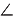AOB = 2APB. The proof can be carried on in three cases:

Case 1: O lies insideAPB

Case 2: O lies onAPB

Case 3: O lies outsideAPBWe shall prove the case 1 here and the rest cases follow on the same lines.

Case 1: Join PO and produce PO to Q. Then OA = OB = OP (radii), so we have two isosceles triangles OAP and OAQ.

LetOAP

= α andOBP = β.

ThenAPO

= α

(base angles of isoscelesOAP)

andBPO

= β

(base angles of isosceles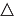OBP).

HenceAOQ

= 2α

(exterior angle ofOAP)

andBOQ

= 2β

(exterior angle ofOBP),AOB

= 2α + 2β = 2(α + β) = 2 ×APB.

The various results parallel to the above result are listed below:

Result: Angle between the tangent and the radius is 90°.

Result: Angles in the same segment are equal.

Result: Angle in a semi-circle is 90°.

Result: Two angles at the circumference subtended by the same arc are equal.

You can get more clarity by viewing the video

Pole and Polar

Let any straight line PAB through the given point P(x1, y1) intersect teh circle S = 0 at two points A and B and if the tangents to teh circle at A and B meet at Q then locus of point Q is called the polar of the point P and the point P is called the pole of the polar with respect to given circle.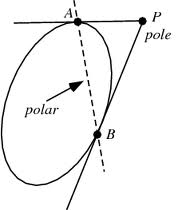Illustration:

The angle between a pair of tangents drawn from a point P to the circle x2 + y2 + 4x - 6y + 9 sin2 + 13cos2 = 0 is 2. The equation of the locus of the point P is

1. x2 + y2 + 4x - 6y + 4 = 0

2. x2 + y2 + 4x - 6y – 9 = 0

3. x2 + y2 + 4x - 6y - 4 = 0

4. x2 + y2 + 4x - 6y + 9 = 0

Solution:

The equation of the circle is x2 + y2 + 4x - 6y + 9 sin2 +13 cos 2 = 0

Hence, the center of the circle = (-2, 3)

Radius of the circle is √ (-2)2 + (32) – 9 sin2 -13cos2

= √13 – 9 sin2 -13cos2

= √13sin2 – 9 sin2

= √4 sin2 = 2 sin

Now, sin  = OA/OP = 2 sin  / √(h+2)2 + (k-3)2

Hence, (h+2)2 + (k-3)2 = 4

h2 + k2 + 4h -6k +9 = 0.

Therefore, the locus of P is x2 +y2 + 4x - 6y +9 = 0.

Illustration:

Tangents drawn from the point P(1,8) to the circle

x2 + y2 - 6x - 4y - 11 = 0 touch the circles at the points A and B. The equation of the circumcircle of the triangle PAB is

1. x2 + y2 + 4x - 6y + 19 = 0

2. x2 + y2 - 4x - 10y + 19 = 0

3. x2 + y2 - 2x + 6y - 29 = 0

4. x2 + y2 - 6x - 4y + 19 = 0

Solution:

The equation of the circle is x2 + y2 - 6x - 4y - 11 = 0

Points P, A, C and B are given to be concyclic as angle PAC + angle PBC = π

Hence, the circumcircle of ?PAB will pass through C.

As angle PAC = π/2, we can use the diametric form where PC is the diameter of the circumcircle of ?PAB.

Using diametric form,

(x-1)(x-3) + (y-8) (y-2) = 0.

x2 + y2 - 4x - 10y + 19 = 0

Illustration:

From the origin chords are drawn to the circle (x-1)2 + y2 = 1. The equation of the locus of the mid-points of these chords is…

Solution:

The given circle is x2 + y2 – x = 0.

Let B (h, k) be the midpoint of a chord OA of the circle x2 + y2 – 2x = 0.

Hence, the equation of the chord bisected at the point is S1 =T.

Hence, h2 + k2 – 2h = xh + yk – (x + h) passes through (0, 0).

So, -h = h2 + k2 -2h

h2 + k2 –h =0.

Hence, the required locus of P is x2 + y2 –x = 0.

Illustration:

Two tangents PQ and PR drawn to the circle x2 + y2 – 2x – 4y – 20 = 0 from point P(16, 7). If the centre of the circle is C then find the area of quadrilateral PQCR.

Solution:

Area of PQCR = 2Δ PQC = 2.1/2 L . r, where L = length of tangent and r = radius of circle.

L = √S1 and r =  √ 1 + 4+ 20 = 5.

Hence, the required area is 75 sq. units.

“Circle” is one of the most scoring and hence an important chapter of Co-ordinate Geometry in the Mathematics syllabus of IIT JEE. There are some fixed patterns of questions asked from this topic. You are expected to do all the questions based on this to remain competitive in IIT JEE examination.

At askIITians we provide you free study material on Co-ordinate Geometry- Circle and you get all the professional help needed to get through IIT JEE.

To read more, Buy study materials of Circles comprising study notes, revision notes, video lectures, previous year solved questions etc. Also browse for more study materials on Mathematics here.### Course Features

• 731 Video Lectures
• Revision Notes
• Previous Year Papers
• Mind Map
• Study Planner
• NCERT Solutions
• Discussion Forum
• Test paper with Video Solution